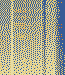## Randomize trials that have already been defined

##### Randomize trials that have already been defined
 Author Message ValerieGroup: Forum Members Posts: 2, Visits: 1 Hello,I have 3 categories of stimuli, containing 5 stimuli each (so, there is a total of 15 stimuli). I want to run 24 trials total, but I do NOT want each category to be presented at equal frequency or ratios. I want the ratio of the stimuli from the three cateorgies to go in this breakdown 7:7:10. How do I do that? In other words, I want to assign a frequency to each category, but then have Inquisit select them at random?They syntax I thought might work is:/trials=random[1-7=noreplace(category1);8-14=noreplace(category2);15-24=noreplace(category3)]However, this does not tell Inquisit to select all the trials randomly because it defines trials 1 through 7 from category1 and trials 8-14 from category 2, etc. I just basically want to tell Inquisit the ratio: category1 should have 7 trials, category2 should have 7 trials, and category3 should have 10 trials (and select the trials in random order following this category ratio).Any suggestions would be greatly appreciated! Thanks! Daveposted 13 Years Ago ANSWERGroup: Administrators Posts: 12K, Visits: 90K To sample trials in the desired proportions (7:7:10), you must simply enter them to the noreplace selection pool in the desired proportions. In your case that's/ trials = [1-24=noreplace(    category1,category1,category1,category1,category1,category1,category1,    category2,category2,category2,category2,category2,category2,category2,    category3,category3,category3,category3,category3,category3,category3,    category3,category3,category3)    ][...]Regards,~Dave ValerieGroup: Forum Members Posts: 2, Visits: 1 Thank you Dave, that was quite helpful!!-Valerie aliciay1Group: Forum Members Posts: 12, Visits: 64 Hi, To add to this question I was wondering if it is possible to manipulate the randomised selection so that no two same stimuli are selected in a row. Is it possible to do this? If so, how would that be established? For example: I have digits 1-9 selected from a list presented 24 times but I want the selection order to be randomised each time and ensure the same digit is not represented twice. Would a no replace function work in this instance?  /items = (trial.practice_go, trial.practice_go, trial.practice_go, trial.practice_go, trial.practice_nogo, trial.practice_go, trial.practice_go, trial.practice_go, trial.practice_go, trial.practice_go, trial.practice_go, trial.practice_go, trial.go, trial.practice_go, trial.practice_go, trial.practice_nogo, trial.practice_go, trial.practice_go, trial.practice_nogo, trial.practice_nogo, trial.practice_go, trial.practice_nogo, trial.practice_go, trial.practice_go)/ replace = falseNote: list of digits used for practice; tied to list.practice_trialtypes/items = (1, 2, 8, 4, 2, 3, 1, 5, 6, 7, 3, 4, 1, 2, 3, 9, 3, 2, 7, 9, 5, 4, 2, 5)/selectionmode = list.practice_trialtype.currentindex/resetinterval = 1/postinstructions = (practiceend MWexamples1 realtask)/trials = [1 = getReadyPrac; 2-25 = list.practice_trialtype]/onblockend = [list.fontsizes.reset()]Apologies if my question is not understandable. Any help would be greatly appreciated.Thanks. Daveposted 3 Years Ago ANSWERGroup: Administrators Posts: 12K, Visits: 90K +x aliciay1 - 5/29/2020Hi, To add to this question I was wondering if it is possible to manipulate the randomised selection so that no two same stimuli are selected in a row. Is it possible to do this? If so, how would that be established? For example: I have digits 1-9 selected from a list presented 24 times but I want the selection order to be randomised each time and ensure the same digit is not represented twice. Would a no replace function work in this instance?  /items = (trial.practice_go, trial.practice_go, trial.practice_go, trial.practice_go, trial.practice_nogo, trial.practice_go, trial.practice_go, trial.practice_go, trial.practice_go, trial.practice_go, trial.practice_go, trial.practice_go, trial.go, trial.practice_go, trial.practice_go, trial.practice_nogo, trial.practice_go, trial.practice_go, trial.practice_nogo, trial.practice_nogo, trial.practice_go, trial.practice_nogo, trial.practice_go, trial.practice_go)/ replace = falseNote: list of digits used for practice; tied to list.practice_trialtypes/items = (1, 2, 8, 4, 2, 3, 1, 5, 6, 7, 3, 4, 1, 2, 3, 9, 3, 2, 7, 9, 5, 4, 2, 5)/selectionmode = list.practice_trialtype.currentindex/resetinterval = 1/postinstructions = (practiceend MWexamples1 realtask)/trials = [1 = getReadyPrac; 2-25 = list.practice_trialtype]/onblockend = [list.fontsizes.reset()]Apologies if my question is not understandable. Any help would be greatly appreciated.Thanks. > I have digits 1-9 selected from a list presented 24 times but I want the selection order to be randomised each time and ensure the same digit is not represented twice. Would a no replace function work in this instance? No, since your digit list's selection is dependent on the selection in the trial type list.Note: list of digits used for practice; tied to list.practice_trialtypes/items = (1, 2, 8, 4, 2, 3, 1, 5, 6, 7, 3, 4, 1, 2, 3, 9, 3, 2, 7, 9, 5, 4, 2, 5)/selectionmode = list.practice_trialtype.currentindex/resetinterval = 1

#### Merge Selected

Merge into selected topic...

Merge into merge target...

Merge into a specific topic ID...